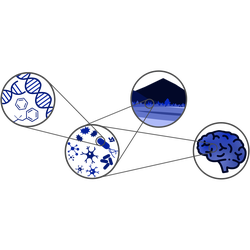View the first lecture here (passcode: K3YNY+Ft).
Topics: Mean field theory for the Ising model and critical behaviors, pt 1 (prof. Amos Maritan)

View the second lecture here (passcode: b7&4+iz0).
Topics: Mean field theory for the Ising model and critical behaviors, pt 2 (prof. Amos Maritan)

View the third lecture here (passcode: PV.903Tb).
Topics: Real-space Renotmalization Group, pt 1 (prof. Amos Maritan)

View the fourth lecture here (passcode: JXu7^3Bh).
Topics: Real-space Renotmalization Group, pt 2 (prof. Amos Maritan)

View the fifth lecture here (passcode: UpuB*5bA).
Topics: Momentum-shell Renormalization Group, pt 1 (prof. Amos Maritan)

View the sixth lecture here (passcode: YD7?0!fR).
Topics: Momentum-shell Renormalization Group, pt 2 (prof. Amos Maritan)

View the seventh lecture here (passcode: 14X^cmS$). Topics: Momentum-shell Renormalization Group, pt 3 (prof. Amos Maritan) View the eight lecture here (passcode: 5C2$?G.g).
Topics: Asymptotic matching for a boundary value problem, dominant balance method (prof. Sandro Azaele)

View the ninth lecture here (passcode: ZA4U%U6%).
Topics: Asymptotic matching for an integral function (prof. Sandro Azaele)

View the tenth lecture here (passcode: &?A%W9&B).
Topics: Boundary layer theory for a boundary value problem (prof. Sandro Azaele)

View the eleventh lecture here (passcode: *%%k8^he).
Topics: Analytical approximations for the solution of a diffusion-advection problem (prof. Sandro Azaele)